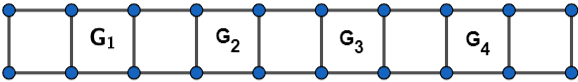QuestionAnswers

# In how many ways can 4 girls and 3 boys be seated in a row so that no two boys are together?Hint:For solving this question, first we will see the important concepts like the fundamental principle of multiplication from permutations and combinations. After that, we will make 4 girls to be seated alternatively in the row and arrange them in $4!$ ways. Then, we will make boys to be seated in the empty spaces between girls and use the formula ${}^{n}{{C}_{r}}=\dfrac{n!}{r!\left( n-r \right)!}$ to get the number of ways in which boys can be seated. Then, we will multiply both results to get the total number of ways possible.

Given:
We have to find the number of ways in which 4 girls and 3 boys be seated in a row so that no two boys are together.
Now, before we proceed we should know the following important concept and formulas:
1. Fundamental Principle of Multiplication: If there are two jobs such that one of them can be completed in $m$ ways, and when it has been completed in any of these $m$ ways, the second job can be completed in $n$ ways. Then, two jobs in succession can be completed in $m\times n$ ways.
2. Number of ways to select $r$ objects from the $n$ distinct objects. The formula for the number of different possible ways is ${}^{n}{{C}_{r}}=\dfrac{n!}{r!\left( n-r \right)!}$ .
3. Number of linear arrangements of $r$ distinct objects will be equal to $r!$ .
Now, first, we will make 4 girls to be seated alternatively in the row. For more clarity, look at the figure given below:In the above figure ${{G}_{1}},{{G}_{2}},{{G}_{3}},{{G}_{4}}$ are the four girls. And we can arrange the four girls in a total $m=4!=24$ number of ways.
Now, if we make boys to be seated in the empty five spaces then, no two boys will be sitting together. And as we know that, we can select $r$ objects from $n$ distinct objects in ${}^{n}{{C}_{r}}=\dfrac{n!}{r!\left( n-r \right)!}$ the number of ways, then we can arrange the boys in $3!$ ways. Then,
Number of ways in which 3 boys can be seated $=n={}^{5}{{C}_{3}}\times 3!=\dfrac{5!}{3!\left( 5-3 \right)!}\times 3!=\dfrac{120}{2}=60$ ways.
Now, from the fundamental principle of multiplication, we can say that the number of ways in which 4 girls and 3 boys be seated in a row so that no two boys are together will be equal to $m\times n=24\times 60=1440$ ways.
Thus, the required number of ways will be $1440$ ways.

Note: Here, the student should first understand what is asked in the question and then proceed with the right approach to get the correct answer quickly. And in such questions of permutations and combinations,Apply the basic concepts and try to visualize the given event through figures. Moreover, for objective type problems, we could have used the formula $\dfrac{\left( g! \right)\left( g+1 \right)!}{\left( g+1-b \right)!}$ directly where $g$ is the number of girls and $b$ is the number of boys.
Group Theory in MathematicsNCERT Book for Class 4 Maths PDF in HindiVariables and Constants in Algebraic ExpressionsNCERT Book for Class 3 Maths PDF in HindiNCERT Books for Class 4 PDF in HindiCBSE Class 11 Maths FormulasCBSE Class 10 Maths Chapter 3 - Pair of Linear Equations in Two Variables FormulaHow to Find Cube Root?NCERT Books for Class 3 PDF in HindiNCERT Book for Class 4 Evs PDF in HindiImportant Questions for CBSE Class 7 Social Science - Social and Political Life Chapter 4 - Growing Up As Boys And GirlsImportant Questions for CBSE Class 11 Maths Chapter 7 - Permutations and CombinationsImportant Questions for CBSE Class 11 Chemistry Chapter 3 - Classification of Elements and Periodicity in PropertiesNCERT Books Free Download for Class 11 Maths Chapter 7 - Permutations and CombinationsImportant Questions for CBSE Class 11 Physics Chapter 4 - Motion in a PlaneImportant Questions for CBSE Class 11 Physics Chapter 3 - Motion in a Straight LineImportant Questions for CBSE Class 7 Science Chapter 11 - Transportation in Animals and PlantsImportant Questions for CBSE Class 8 Social Science Our Past 3 Chapter 4 - Tribals, Dikus and the Vision of a Golden AgeImportant Questions for CBSE Class 8 Social Science Our Past 3 Chapter 1 - How, When and WhereImportant Questions for CBSE Class 11 Maths Chapter 4 - Principle of Mathematical InductionCBSE Class 12 Maths Question Paper 2020CBSE Class 10 English Language and Literature Question Paper with SolutionsEnglish Language and Literature Question Paper for CBSE Class 10 - 2012English Language and Literature Question Paper for CBSE Class 10 - 2010English Language and Literature Question Paper for CBSE Class 10 - 2011CBSE Class 10 Maths Question Paper 2020CBSE Class 10 Maths Question Paper 2017Maths Question Paper for CBSE Class 10 - 2011Maths Question Paper for CBSE Class 10 - 2008Maths Question Paper for CBSE Class 10 - 2012NCERT Solutions for Class 11 Maths Chapter 7RD Sharma Class 11 Solutions Chapter 3 - Functions (Ex 3.4) Exercise 3.4NCERT Solutions for Class 11 Maths Chapter 3 Trigonometric Functions (Ex 3.4) Exercise 3.4NCERT Solutions for Class 11 Maths Chapter 7 Permutations and Combinations in HindiRS Aggarwal Solutions Class 10 Chapter 4 - Triangles (Ex 4C) Exercise 4.3RD Sharma Class 8 Solutions Chapter 4 - Cube and Cube Roots (Ex 4.3) Exercise 4.3RD Sharma Class 7 Solutions Chapter 4 - Rational Numbers (Ex 4.3) Exercise 4.3RS Aggarwal Solutions Class 7 Chapter-3 Decimals (Ex 3D) Exercise 3.4RS Aggarwal Solutions Class 8 Chapter-3 Squares and Square Roots (Ex 3D) Exercise 3.4RD Sharma Class 10 Solutions Chapter 4 - Exercise 4.3